Next: Other uses of the Up: Subset-Sum (Knapsack) problems and Previous: A proposed public-key system   Contents

## Breaking Knapsack Cryptosystems

We will present some of the basic ideas for attacking the system described in the preceding section, and illustrate them on a small example.4 Our source is the paper by E. Brickell and A. Odlyzko, Cryptanalysis: a survey of recent results,'' in Contemporary cryptology: the science of information integrity.

It is not necessary to provide an efficient algorithm guaranteed to crack all instances of a cryptosystem to call its security into question, and our analysis is only an indication that the simple system of the previous section can often be broken.

Suppose we are given the sequence:generated using an unknown multiplier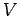(correponding to 1000 in the previous example), an unknown modulus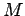, and an unknown super-increasing sequence. Let. Then there are non-negativesuch that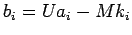.must be larger than all the, hence it must be significantly larger than the earliest members of the sequence.

The decryption method begins by guessing which of the knowncorrespond to a few of the early members of the sequence. A trial-and-error approach is considered feasible for practical size problems.

Suppose we have guessed that 2464, 611, and 2456 correspond to the first three, and further that 2464 corresponds to.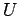may be eliminated from pairs of equations to obtain:Sinceis large compared to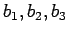this impliesmust be small-- about the size of(positive or negative). In other words,andmust both be close to 0 or to 2464 mod 2464.

It is plausible that restrictions of this kind give a lot of information about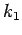. As we look at all possible values of,mod 2464 is evenly distributed through the entire range from 0 to 2463. If we require, for example, that the number is eitheror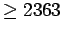, we would expect that only aboutof the values forsatisfy this. It seems plausible that the distribution ofis independent of the distribution of, so a similar restriction on the second number reduces the possible values ofby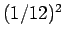.

In our example,implies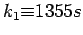and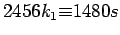. We look at the values of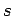for something which makesmod 2464 small. Part of the results:The multiples ofare clearly the most likely candidates. We try, which gives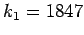.

[The published algorithm recommends guessing more than 3 of the lowestand determiningfrom an integer program: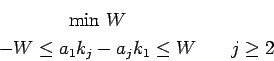using a special algorithm which is polynomial-time for a fixed number of constraints.]

Sinceimpliesis close to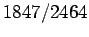, we try using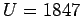,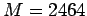on the, which gives: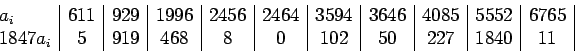This is not a super-increasing sequence, since. However, it is close enough to one that one can use it to solve subset-sum problems with a small amount of work.

Thewere generated using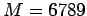,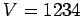,. Comparison of the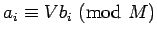with the almost'' super-increasing sequence obtained above shows that, except for the first few terms, the ratio of terms from the two sequences is nearly constant. Since a multiple of a super-increasing sequence is super-increasing, this explains why we would expect to obtain a useful sequence.#### Footnotes

...4
Calculations in this section were done using the Calc program based on the emacs editor. Both programs are available free as part of the GNU system.Next: Other uses of the Up: Subset-Sum (Knapsack) problems and Previous: A proposed public-key system   Contents
Translated from LaTeX by Scott Sutherland
2002-12-14